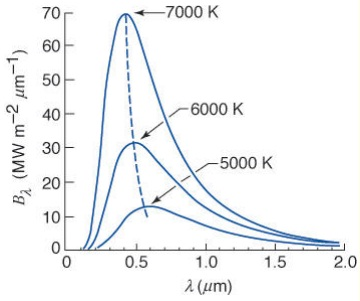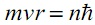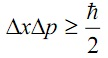#### Inadequacies of Classical Mechanics, Physics tutorial

Introduction:

If classical methods had no inadequacies, there would have been no requirement for a new theory. Up until the turn of the century, it was thought that the Newton's laws could account for all the physical phenomena, irrespective of the size of the particle comprised, and for any particle travelling at whatsoever speed.

As till now, you are familiar by the fundamental ideas of classical methods, based on the Newton's laws of motion. It would come out that once you are familiar that the equation of motion of a body, you can concurrently and precisely predict its position and linear momentum at any other time. Furthermore, you would anticipate that an electron confined in the walls of a finite potential well, provided the energy is less than the height of the well, would encompass no effect outside the borders of the well. Obviously, the harmonic oscillator you came across could encompass zero energy.

Everybody at a temperature higher than the absolute zero radiates energy as the internal energy (or thermal) is transformed to electromagnetic radiation. The spectrum of frequencies emitted through the body based on its temperature. Therefore, at low energies, the lower frequencies (or longer wavelengths) are predominant. At higher temperatures, the higher frequencies (that is, shorter wavelengths) are more prominent. This would be seen that the peak of the spectrum of radiation moves in the direction of the higher frequencies as the temperature of the body increases.

A blackbody is the perfect absorber and emitter of the radiation. An illustration of a blackbody would be a small hole in the heated cavity. Radiation falling on such a hole would be bounced up and down within the cavity, due to reflection, till all the radiation is absorbed.

Prior to the time of Planck, Stefan and Boltzmann came up by a formula for determining the total radiant energy emitted through a blackbody per unit surface area per unit time, as:

j* = σT4

Here 'σ' is the Stefan-Boltzmann constant, equivalent to 5.6704 x 10-8 Js-1m-2K-4 and T is the absolute temperature of the body. By using this law, Stefan was capable to find out the temperature of the sun.

Rayleigh and Jeans predicted, based on the principle of equipartition of the energy that the radiation emitted through a blackbody per unit time, per unit area is represented as:

u = 8πv2kT/c3

Here 'k' is the Boltzmann constant, 'v' is the frequency of the radiation and 'T' is the temperature of the body.

Though, neither the Stefan-Boltzmann nor the Rayleigh-Jeans formula could provide the true picture (that is, experimental); that is, neither fitted the observed spectrum of radiation. The former agreed by the observation at high frequencies whereas the latter was quite good for low frequencies.

By making the supposition that radiant energy could merely be emitted or absorbed in the quanta (or little packets of energy), Planck comes out at the formula:

u = (8πh/c3) [v3/(ehv/KT) -1]

The above formula agrees by the observation at all frequencies. The figure represents the spectrum for three temperatures: 5000 K, 6000 K and 7000 K.Photoelectric effect:

Photoelectric effect is a phenomenon in which the electrons are emitted from the matter due to irradiation through electromagnetic waves of appropriate frequency.

Classical mechanics predicts that the higher the intensity of the incident wave, the higher the velocity of the emitted electron. Furthermore, it expects any frequency of light to emit the electrons. This was, though, not observed in nature. To begin with, the electrons were never emitted unless the frequency of the incident wave was a certain value. In another words, there is a threshold beneath which the incident radiation wouldn't emit any electrons.

Einstein supposed that the incident radiation comprised of little packets of energy, quanta of light, termed as photons, each of energy E = hf, here 'h' is the Planck's constant and 'v' is the frequency of the incident radiation.

The supposition was that an electron would either absorb a photon or not. In the event that the electron absorbs this energy, then, portion of the energy would be employed in overcoming the work function of the metal, that is, the energy binding the electron to the metal. The surplus energy would then be available for the electron to move away from the metal. In another words, if the energy of the photon is just adequate to overcome the work function, the electron would be emitted, however wouldn't encompass adequate energy to leave the surface of the metal.

Therefore, the formula guiding photoelectric effect is as follows:

E = hf = Φ + Kmax = Φ + 1/2 mv2max

Here, Kmax is the maximum kinetic energy of the emitted electron. It obeys that the maximum velocity an emitted electron can encompass is:

vmax = √2(hf - Φ)

This is clear from the above equation, that for E = hf less than the work function, no electron is emitted, meaning that, since 'h' is a constant, if the frequency, 'f', is less than the threshold, no electron is emitted. Therefore, the maximum kinetic energy a photon can encompass, hf - Φ is independent of the intensity of the radiation, being a function just of the frequency; given the metal remains the similar (that is, the work function Φ remains constant).

We can as well write the work function Φ as Φ = hfo, here 'fo' is the threshold frequency, beneath which no electron will be emitted. We can thus now represent:

v = √2h (f - fo)

From the above equation, it is obvious that no electron will be emitted when f < fo the threshold frequency.

For a given metal and a given radiation, the intensity of the radiation is directly proportional to the number of electrons emitted. This is due to the reason that more electrons would be exposed to the radiation.

The experimental set-up for photoelectric effect comprises of an emitter plate and a collector plate. What opposing potential would stop the most energetic electrons from arriving the opposite plate of the collector?

1/2 mv2max = eVo

Here 'e' is the charge on the electron and Vo is the stopping potential, as the potential energy just balances the kinetic energy. Solving for Vo

Vo = mv2max/2e

Compton Effect:

Let a photon having wavelength 'λ' collide by an electron at rest. After the collision, the photon is scattered in the direction 'θ' relative to the initial direction. The electron is as well scattered at an angle 'Φ' with respect to the reference direction. We shall apply relativistic conditions, meaning that the speeds comprised are large adequate to be considered relativistic.

Conservation of energy:

E = Ee = E' + Ee'

hv + mec2 = hv' + √[Pe2c2 + (mec2)2]

From the relativistic energy-momentum relation:

Ee2 = Pe2c2 + (mec2)2

Conservation of linear momentum:

P + Pe = P' + Pe

However, Pe = 0 therefore,

P = P' + Pe'

From equation:

hv + mec2 = hv' + √[Pe2c2 + (mec2)2]

On solving, we get:

c/v - c/v' = (h/mec) (1 - cos θ)

As c/v = λ and c/v' = λ'. We can thus write:

λ - λ' = h/mec (1 - cos θ)

Bohr's Theory of the Hydrogen Atom:

Rutherford attempted to describe the structure of the atom through proposing a central nucleus, by the electrons moving in orbits round the nucleus. Though, quite a number of things were not right with his model. For illustration, you would have been taught before now, that an accelerating charge would radiate energy. Keep in mind that a body on curvilinear motion is accelerating even if the magnitude of the velocity remains constant (that is, centripetal acceleration). As such, you would anticipate an electron moving round the nucleus to lose energy and afterward fall into the massive nucleus. We thus would have expected a continuous spectrum of energy as against the line spectra obtained in the reality. Bohr tried to resolve this problem through making a few postulates:

1) An electron can merely orbit the nucleus at some radii, such that the condition:Here all the symbols have their standard meanings. Therefore, the radii are quantized; not just any radius would do. Whereas in such an orbit, the electron would not radiate energy.

2) Electrons can form a transition from one allowable orbit (or correspondingly, energy level) to the other via absorbing or emitting the difference in energy, as the case might be. That is, the energy difference is:

Ef - Ei = hv

Here 'v' is the frequency of the emitted photon, Ei and Ef being the initial and the final energy levels correspondingly.

Taking into concern the Coulombic force between the proton and the electron in the hydrogen atom, Bohr appeared at the allowable radii of the form:

rn = Cn2

Here 'C' is a constant. Likewise, the energy levels are as well of the form:

En = D/n2

Here 'D' is a constant.

Wave-particle duality:

So far, we have concern the radiant energy and photons as being quantized. On the other hand, according to the de-Broglie in the year 1924, matter consists of a wave nature, by the wavelength represented by:

h = pλ

The above formula was afterward confirmed experimentally through electron diffraction. Other phenomena which support the wave nature of light comprise refraction, diffraction and the interference.

From the prior, it is quite obvious that matter behaves as if composed of particles or as a wave, therefore the word wave-particle duality.

Heisenberg's Uncertainty Principle:

Classical physics states to recognize the future state of any particle, given the position and the momentum are known at any point. Heisenberg's uncertainty principle prohibits the possibility of concurrently measuring the position and the linear momentum of any object with infinite accuracy. Certainly, any attempt to measure one of the quantities by infinite accuracy leads to infinite uncertainty in the other. In fact, if the uncertainty in position Δx and that of the linear momentum of the particle is Δp, then,Therefore, our whole world of classical mechanics appears to have melted away. Though, classical mechanics has been tested and trusted for so long. Now the question arises why does it now seem to fail? This is due to the reason that we have been dealing by the matter on the large scale. Quantum mechanics makes the difference if we have to deal with small objects, for example on the scale of atoms. However, quantum mechanics has to agree by classical physics in certain limits, in conformity by the correspondence principle.

Tutorsglobe: A way to secure high grade in your curriculum (Online Tutoring)